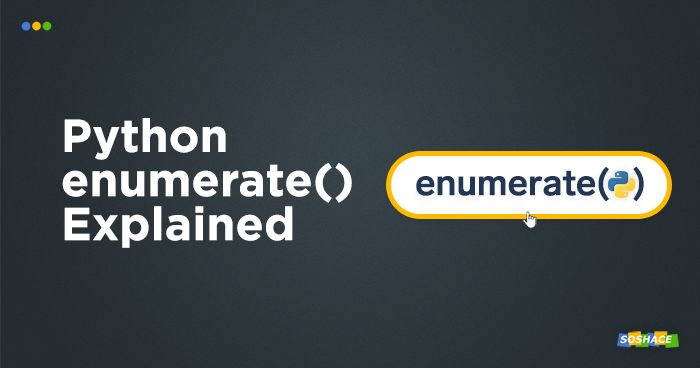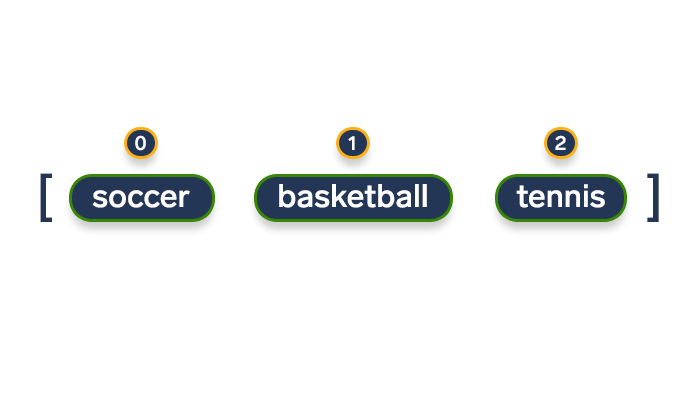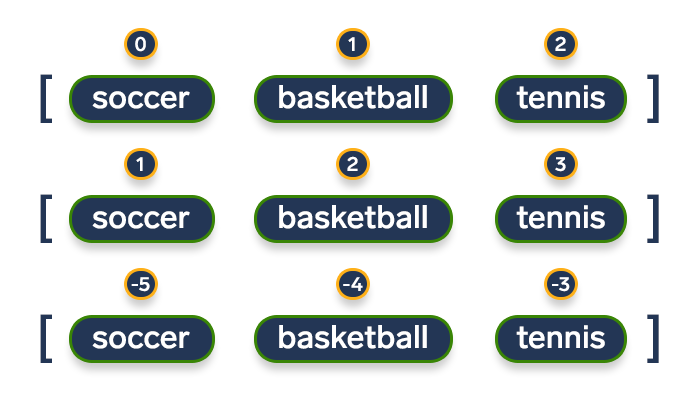# Python enumerate() Explained and VisualizedExplaining Python 3, episode 1

Python is the favorite programming language of many developers; contrary to popular belief, it isn’t merely a “technology for newbies” — its power and functionality make it an excellent tool for a plethora of tasks (our Python interview questions prove it quite well)

Python is renowned for its collection of libraries, modules, and functions that it comes packaged with — and enumerate() is something many developers want to understand better. In this article, we’ll explore what enumerate() actually is, analyze its functionality, and highlight how you should use it to maximize its efficiency and performance. Read enough of our articles — and you might be able to join our platform and find great remote projects to work on!## OK, so what does enumerate() do?

In many situations, we need to get the element’s index while iterating over an iterable element (i.e. any object that we can loop over). One way to achieve the desired result would be this:

```animals = ['dog', 'cat', 'mouse']
for i in range(len(animals)):
print(i, animals[i])```

which will result in:

```0 dog
1 cat
2 mouse```

Most developers coming from a C++/Java background will probably opt for the implementation above — iterating over the iterable’s length via the index is a familiar concept for them. The problem with this approach, however, is its inefficiency. `enumerate()` is the right way to do things:

```for i, j in enumerate(example):
print(i, j)```

As revealed by Python’s in-built help() module, The enumerate object yields pairs containing a count (from start, which defaults to zero) and a value yielded by the iterable argument. Another description can be found in Allen B. Downey’s excellent Think Python book: The result from enumerate is an enumerate object, which iterates a sequence of pairs; each pair contains an index (starting from 0) and an element from the given sequence.

enumerate() offers a great functionality — for instance, it comes in handy when you need to obtain an indexed list:

`(0, seq), (1, seq), (2, seq), ...`

## Case study 1: Enumerate a stringA string is just a list, metaphorically speaking

To understand string enumeration better, we can imagine our given string as a collection of individual characters (items). Therefore, enumerating over a string will give us:

1. The character’s index.
2. The character’s value.
```word = "Speed"
for index, char in enumerate(word):
print(f"The index is '{index}' and the character value is '{char}'")```

And here’s the output:

```The index is '0' and the character value is 'S'
The index is '1' and the character value is 'p'
The index is '2' and the character value is 'e'
The index is '3' and the character value is 'e'
The index is '4' and the character value is 'd'```

## Case study 2: Enumerate a listSo how should we go about enumerating a list?..

The next logical step is enumerating a list. In order to do this, we can utilize a `for` loop and iterate over each item’s index and value:

```sports = ['soccer', 'basketball', 'tennis']
for index, value in enumerate(sports):
print(f"The item's index is {index} and its value is '{value}'")```

The output will be:

```The item's index is 0 and its value is 'soccer'
The item's index is 1 and its value is 'basketball'
The item's index is 2 and its value is 'tennis'```

## Case study 3: Customizing the starting indexStarting index can be changed

We can see that enumeration begins at the index 0; however, we often need to change the starting position to allow for more customizability. Thankfully, enumerate() also takes an optional argument [start]

`enumerate(iterable, start=0)`

which can be used to indicate the index start position. Here’s how you can implement it:

```students = ['John', 'Jane', 'J-Bot 137']
for index, item in enumerate(students, start=1):
print(f"The index is {index} and the list element is '{item}'")```

This gives us…

```The index is 1 and the list element is 'John'
The index is 2 and the list element is 'Jane'
The index is 3 and the list element is 'J-Bot 137'```

Now let’s edit the code a bit – you’ll see that:

• The starting index can be negative.
• You can omit `start=` and simply pass the integer argument without it.
```teachers = ['Mary', 'Mark', 'Merlin']
for index, item in enumerate(teachers, -5):
print(f"The index is {index} and the list element is '{item}'")```

The output will be:

```The index is -5 and the list element is 'Mary'
The index is -4 and the list element is 'Mark'
The index is -3 and the list element is 'Merlin'```

## Case study 4: Enumerate a tuple

Working with tuples follows the same logic as list enumeration:

```colors = ('red', 'green', 'blue')
for index, value in enumerate(colors):
print(f"The item's index is {index} and its value is '{value}'")```

The output:

```The item's index is 0 and its value is 'red'
The item's index is 1 and its value is 'green'
The item's index is 2 and its value is 'blue'```

## Case study 5: Enumerate a list of tuplesTuples in lists, in tuples, in lists, in…

Let’s take it up a notch and combine a number of tuples into a list… and now we want to enumerate this list of tuples. One option would be writing code like this:

```letters = [('a', 'A'), ('b', 'B'), ('c', 'C')]
for index, value in enumerate(letters):
lowercase = value
uppercase = value
print(f"Index '{index}' refers to the letters '{lowercase}' and '{uppercase}'")```

However, tuple unpacking proves to be a more efficient approach. Here’s an example:

```letters = [('a', 'A'), ('b', 'B'), ('c', 'C')]
for i, (lowercase, uppercase) in enumerate(letters):
print(f"Index '{i}' refers to the letters '{lowercase}' and '{uppercase}'")```

This will output:

```Index '0' refers to the letters 'a' and 'A'
Index '1' refers to the letters 'b' and 'B'
Index '2' refers to the letters 'c' and 'C'```

## Case study 6: Enumerate a dictionaryThe English — Integer Dictionary

It may seem that enumerating a dictionary would be similar to enumerating a string or a list — but it’s not. The main difference associated with dictionaries is their order structure, i.e. the way the elements are ordered in this particular data structure. Dictionaries are somewhat arbitrary because the order of their items is unpredictable. If we create a dictionary and print it, we’ll get one order:

```translation = {'one': 'uno', 'two': 'dos', 'three': 'tres'}
print(translation)
# Output on our computer: {'one': 'uno', 'two': 'dos', 'three': 'tres'}```

However, if you print this very dictionary, the order might be different!

As dictionary items cannot be accessed by indices, we’ll have to utilize the `for` loop to iterate over the dictionary’s keys and values. The `key — value` pair is called an item, so we’ll use the `.items()` method for this purpose:

```animals = {'cat': 3, 'dog': 6, 'bird': 9}
for key, value in animals.items():
print(key, value)```

The output will be:

```cat 3
dog 6
bird 9```

## Conclusion

We’ve got numerous other Python 3 functions, libraries, and modules to cover in the future — stay tuned!The post Python enumerate() Explained and Visualized appeared first on Soshace.

Source: Soshace